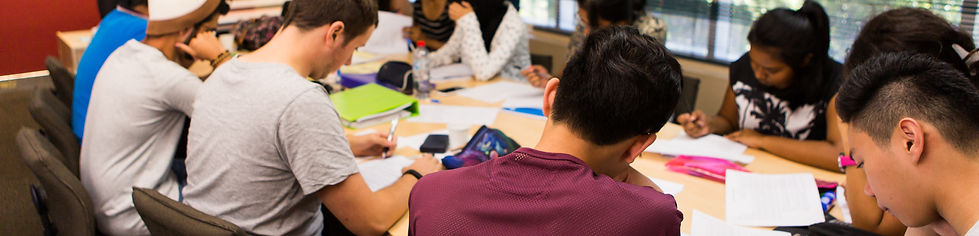Year 12 HSC
Mathematics (General)

By the end of Year 12, students will be able to use two-dimensional and three-dimensional models to solve practical problems and understand the relationship between changing quantities in algebraic and graphical form. Students will be able to solve and apply Pythagoras’ theorem and trigonometric ratios to trigonometric problems. They will be able to model financial situations and represent collected data appropriately. Students will be able to use statistics and analyse data to make inferences, predictions and conclusions. They will be able to make predictions based on mathematical models and about financial situations. Students can use results of measurements and calculations to make judgements about reasonableness. Students can apply mathematical techniques to real life through mathematical argument and reasoning.

Timetable
Timetable

By the end of Year 12, students are able to solve and sketch algebraic functions and inequalities involving rational numbers, surds, indices, quadratics and cubics, simple algebraic fractions and absolute functions. They use trigonometric ratios and rules, can calculate the area of non right-angled triangles and sketch trigonometric functions. Students apply deductive reasoning to proofs and numerical exercises involving plane figures. Students are able to use rules of differentiation and integration and apply calculus geometrically as in rates of change and exponential growth and decay. They list outcomes for multi-step chance experiments and assign probabilities for these experiments.